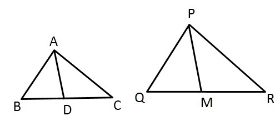# SideAB and BC and median AD of a triangle ABC are respectively proportional to sides PQ and QR and median PM of ?PQR (check image). Show that ΔABC ~ ΔPQR•August 17, 2020

Given two triangles, ΔABC and ΔPQR in which AB, BC and median AD of ΔABC are proportional to sides PQ, QR and median PM of ΔPQR

To Prove: ΔABC ~ ΔPQR

We have? AB/PQ = BC/QR = AD/PM (D is the mid-point of BC. M is the midpoint of QR)

ΔABD ~ ΔPQM [SSS similarity criterion]

Therefore, ∠ABD = ∠PQM [Corresponding angles of two similar triangles are equal]

∠ABC = ∠PQR

In ΔABC and ΔPQR

AB/PQ = BC/QR ———(i)

∠ABC = ∠PQR ——-(ii)

From the above equation (i) and (ii), we get

ΔABC ~ ΔPQR [By SAS similarity criterion]

Comment